• WORK
• TOOLS
• TRAINING
• VIEWS
• CONTACT

This is the third in a series of blog posts where I illustrate the use of Excel as an aid to the study of calculus. I've already published a blog post about graphing functions with a single independent variable x in a cartesian coordinate system and a blog post about plotting parametric equations. In this post we'll be looking at plotting 2D polar equations.

Up to this point we have studied curves in the plane in which the position of the points is represented by cartesian coordinates (x,y). There is an additional way to describe such curves by their polar coordinates (r,𝜃) where r measures the radial distance from the origin along a ray that makes an angle 𝜃 with some fixed reference ray (usually the positive x axis).

However, we already know that there is a relationship between the two systems as shown below:

Next, I need to generate a collection of ordered pairs (r,𝜃). For that I'm going to use Excel's new Dynamic Arrays functionality to spill a series of 10 equally spaced 𝜃s using the SEQUENCE function as follows:

5. Enter the following formula in cell A8
= SEQUENCE(C4,1,C2,C5)

The worksheet should look like this:

# MATHS IN EXCEL - 2D POLAR PLOTS

6. Enter the following formula in cell B8:
= IFERROR(EXP(COS(A8#)) - 2 * COS(4 * A8#) + (SIN(A8# / 12))^5,NA())

7. Enter the following formulas in cells C8 and D8 respectively:
C8 --> = B8# * COS(A8#)

D8 --> = B8# * SIN(A8#)

Notice that I used the IFERROR function to force an #N/A where functions cannot be evaluated (i.e. division by zero, LN of numbers less than or equal to zero, square roots of negative numbers, etc.). Like that the Excel charts will show #N/A as an empty cells (gaps) instead of a zero.

The worksheet should look like this:

Next, lets create two named ranges—one for the Xs and one for the Ys—that will be used as data ranges for the chart.

8. On the Formulas tab, in the Defined Names group, click Define Name.
9. In the New Name dialog box, in the Name box, type lstX.
10. In the Refers to box, click the Collapse Dialog button (which temporarily shrinks the dialog box), select cell C8 on the worksheet, type # at the end of the reference and then click the Expand Dialog as follows:

11. Click the Ok button to close the New Name dialog box.
12. Repeat steps 8-11 to create another named range lstY by referring to cell D8 (don't forget to add the hash key # at the end of reference to tell Excel that you are referencing a Dynamic Array).

By now we have created two named ranges lstX and lstY as can be seen in the Name Manager dialog box below:

13. Next, click Insert > Scatter > Scatter with Smooth Lines to insert a scatterplot.
14. On the worksheet, right-click the chart and then, on the shortcut menu, click Select Data.
15. Next, In the Select Data Source dialog box, add a new data series by clicking the Add button under Legend Entries (Series).
16. In the Edit Series dialog box, type "Function" in the Series name edit box.
17. Next, edit the Series X values to refer to cell C8.
18. Delete \$C\$8 from the newly created reference so that you end up with =Sheet1! in the box.
19. Next, press F3. The Paste Name dialog box appears. Select lstX from the Paste name list and press the Ok button to close the Paste Name dialog box.
20. Repeat steps 17-19 above to edit the Series Y values by referring to lstY as per below:

21. Press the Ok button twice in a row to close the Edit Series and the Select Data Source dialog boxes and the chart should look like this:

So far we've used 10 data points to draw the curve. To increase the accuracy of the plot lets increase the # of points.

22. In cell C4 type 600.

Because we used Dynamic Arrays in cells C8 and D8 the calculations will spill to generate 600 pairs of data points. Since we used Named Ranges, the chart will automatically adjust and should look like this:

Lets graph the function

r(𝜃) = ecos(𝜃) - 2 * cos(4𝜃) + sin5(𝜃/12)

where 𝜃 ranges through the domain [0,75.4]

The first thing to do is to define the boundaries of the 𝜃 interval, the number of points to be generated and the step between each point measured by the angle around the origin of the Pole.

1. Enter "Lower bound", "Upper bound", "# of points" and "Step" in cells B2, B3, B4 and B5 respectively.
2. Enter the number 0 in cell C2 and the number 75.4 in cell C3.
3. Enter the number 10 in cell C4. This will define the number of points that will make up the graph. The more points we have the more accurate the shape of the curve will be (more about this later).
4. Enter the following formula in cell C5
= (C3 - C2) / (C4 - 1)

The worksheet should look like this:

Views > Excel charts > Maths in excel 2D polar plots

MATHS IN EXCEL - 2D POLAR PLOTS

23. Finally, do some formatting such the color of curve, the font, its color and size, and gridlines. Add chart title and the equation and the graph should look like this:

24. If you want to plot the curve of another equation, say r(𝜃) = tan(17𝜃) + cot(17𝜃) in the interval [0,10.1], then type the following formulas in cells B8:
= IFERROR(TAN(17 * A8#) + COT(17 * A8#),NA())

25. Type 0 in cell C2 and 10.1 in cell C3 and 500 in cell C4.

Adjust the boundaries of the horizontal and vertical scales and the chart should look like this: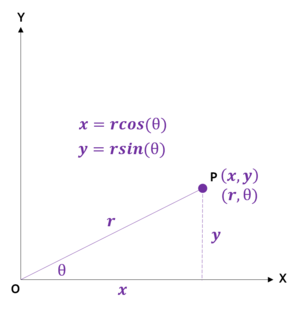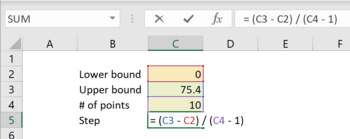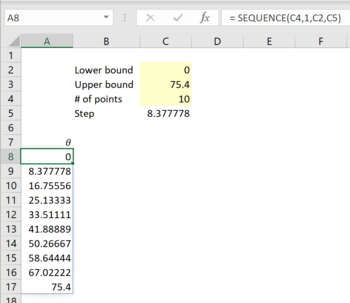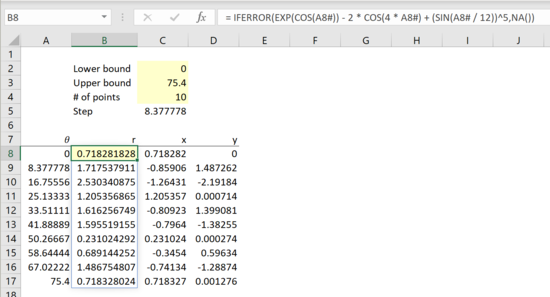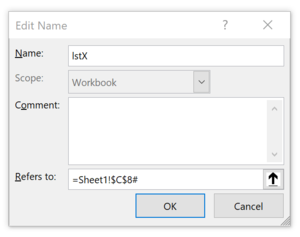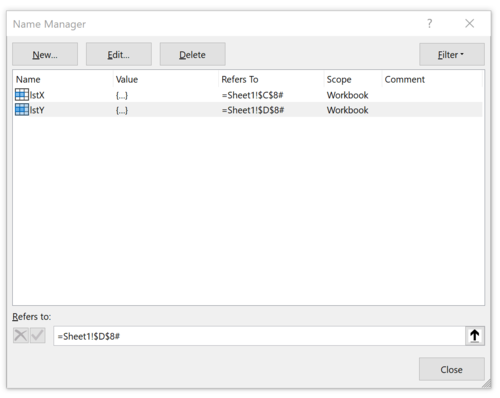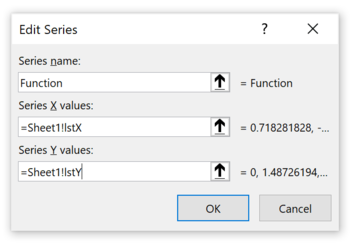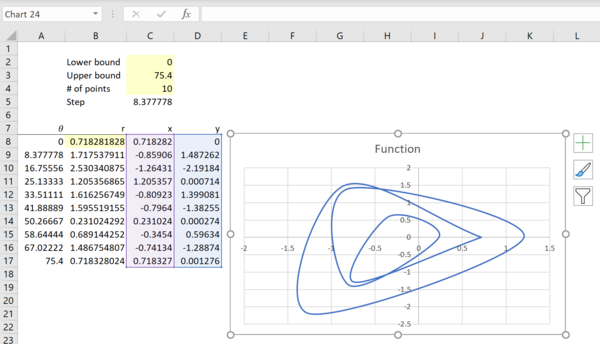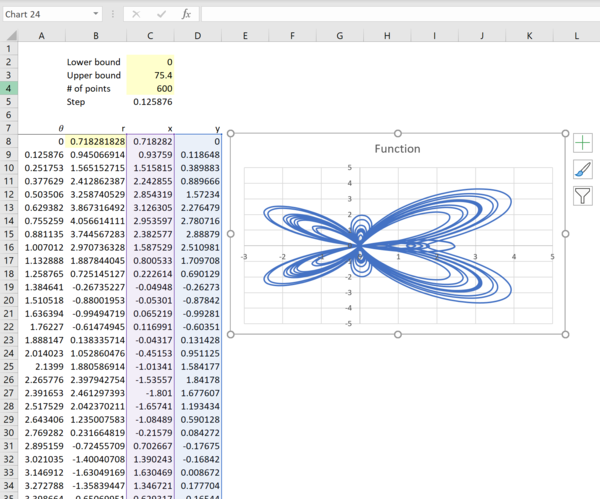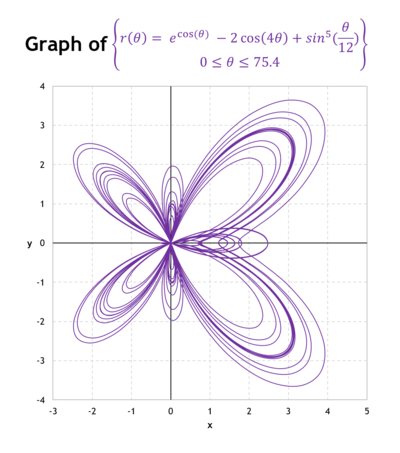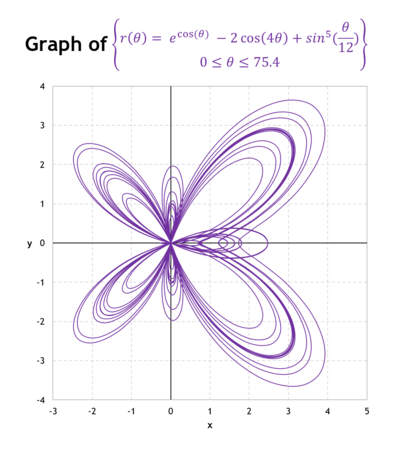Thank you for reading, and I hope you found this useful. If you have any questions, find me on Twitter and ask me anything.